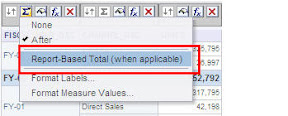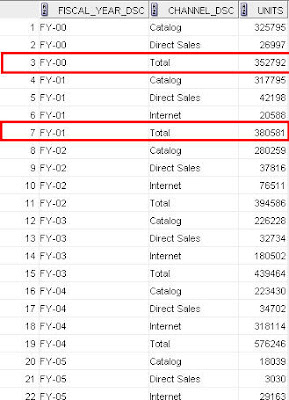# Archive for May 16th, 2009

## Oracle BI EE 10.1.3.4.1 – Report SUM and Pivot Calculations – An alternative SQL on Oracle 10g and above – Equivalence to MDX – MODEL Clause – Part 2

Posted by Venkatakrishnan J on May 16, 2009

One of the few things that was discussed in the BI Forum was the fact that BI EE sometimes generates absolutely un-necessary queries while using a custom Report Sum. For example, if you look at the report below, you would notice that we have added a custom summation row after every Year to get the Year wise sub-totalsThere are 2 ways of producing this summation. One is by making the BI Server to fire a query back to the database (which would generate the sum) and the other is by letting the BI Server itself to produce the sum. Lets first choose the first option by disabling the report based totals(making BI EE to generate the sub-total query as well).If you look at the query you would notice that the query generated by BI EE does not actually produce the result in the actual desired format. The query is given below

```select
distinct D1.c2 as c1,
D1.c3 as c2, D1.c1 as c3,
sum(D1.c1) over () as c4
from
(
select sum(T10976.UNITS) as c1,
T10939.FISCAL_YEAR_DSC as c2,
T10881.CHANNEL_DSC as c3
from
CHANNEL_DIM T10881,
TIME_DIM T10939,
UNITS_FACT T10976 where ( T10881.CHANNEL_ID = T10976.CHANNEL_ID and T10939.MONTH_ID = T10976.MONTH_ID )
group by
T10881.CHANNEL_DSC,
T10939.FISCAL_YEAR_DSC ) D1
order by c1, c2
```

This query would not generate the data in the desired format(instead of new summation row, this would generate a new column that BI Server would have to manipulate). So, this begs the question of how do we generate a sql which produces an output exactly same as the above report. This in itself is a nice sql challenge as it is not possible out of the box using normal sql constructs. This is where MODEL clause can come to our rescue. In the last blog entry on this topic, i had shown you how to go about generating AGO and ToDate functions using direct sql on the summed up result set instead of the base tables. In the same fashion, MODEL clause can be used to generate custom sub totals. For example, the same query above can be re-written as shown below

```SELECT FISCAL_YEAR_DSC, CHANNEL_DSC, UNITS FROM (select sum(T10976.UNITS) as UNITS,
T10939.FISCAL_YEAR_DSC as FISCAL_YEAR_DSC,
T10881.CHANNEL_DSC as CHANNEL_DSC
from
CHANNEL_DIM T10881,
TIME_DIM T10939,
UNITS_FACT T10976
where  ( T10881.CHANNEL_ID = T10976.CHANNEL_ID and T10939.MONTH_ID = T10976.MONTH_ID )
group by T10881.CHANNEL_DSC, T10939.FISCAL_YEAR_DSC)
MODEL
PARTITION BY (FISCAL_YEAR_DSC)
DIMENSION BY (CHANNEL_DSC)
MEASURES (UNITS)
RULES (UNITS['Total'] = SUM(UNITS)[ANY])
ORDER BY 1,2
```In order to use MODEL, we would have to understand certain basics of how it can be used and in what situations it can be used. MODEL was introduced to generate sql queries that enables an end user to visualize the data in a multi-dimensional format. One can generate Multi MODEL references to do currency restatements etc. To understand this lets look at the BSO architecture diagram of Essbase. As you would probably know, BSO option consists of 2 parts

1. Sparse Dimensions that are stored as an index – So, these dimensions are typically stored in a normal relational row-wise format in Essbase.
2. Dense Dimensions that are stored as an Multi-Dimensional Array – These dimensions form a multi-dimensional array. The pointer to the array is denoted by the corresponding sparse dimension combinationIn the same way, a MODEL clause consists of an index denoted by the Partition By Clause. The multidimensional array or a Block is denoted by the DIMENSION clause. The only difference between the 2 is the fact that you would have multiple measure blocks per partition by clause dimension combination. In the case of essbase, an index entry points to only one block. The reason for this is, MODEL clause does not treat the measure values as a seperate dimension. So, a MODEL clause treats a summarized sql query as shown belowIf you understand this analogy, it would be easier to understand the analogy between Essbase member formulas/calculation scripts to the MODEL RULES clause. I would go into detail of how these 2 are related in a future blog entry. For now, lets take another pivot table example as shown below.As you see, the above is a very simple pivot table containing a calculated row (DIrect Sales+Catalog). If you look at the query generated by BI EE, you would notice, that BI Server would be doing this calculation in memory. Now, our aim is to generate the pivot table report with the calculation too in a single query using MODEL clause

```select T10939.FISCAL_YEAR_DSC as c1,
T10881.CHANNEL_DSC as c2,
sum(T10976.UNITS) as c3
from
CHANNEL_DIM T10881,
TIME_DIM T10939,
UNITS_FACT T10976
where  ( T10881.CHANNEL_ID = T10976.CHANNEL_ID and T10939.MONTH_ID = T10976.MONTH_ID )
group by T10881.CHANNEL_DSC, T10939.FISCAL_YEAR_DSC
order by c1, c2
```

In order to do that, we would have to generate a rule that would produce a new set of rows in addition to the existing rowset.

```SELECT CHANNEL_DSC, FISCAL_YEAR_DSC,  UNITS FROM (select sum(T10976.UNITS) as UNITS,
T10939.FISCAL_YEAR_DSC as FISCAL_YEAR_DSC,
T10881.CHANNEL_DSC as CHANNEL_DSC
from
CHANNEL_DIM T10881,
TIME_DIM T10939,
UNITS_FACT T10976
where  ( T10881.CHANNEL_ID = T10976.CHANNEL_ID and T10939.MONTH_ID = T10976.MONTH_ID )
group by T10881.CHANNEL_DSC, T10939.FISCAL_YEAR_DSC)
MODEL
PARTITION BY (FISCAL_YEAR_DSC)
DIMENSION BY (CHANNEL_DSC)
MEASURES (UNITS)
RULES (UNITS['Calculated'] = SUM(UNITS)[ANY])
ORDER BY 1,2
```As you see, MODEL clause has quite a few advantages whenever we have data manipulations on a summarized result. We shall see more complex examples in a future blog entry.